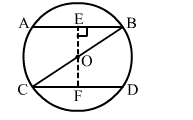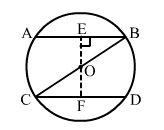# In the given figure, BOC is a diameter of a circle with centre O.

Question:

In the given figure, BOC is a diameter of a circle with centre O. If AB and CD are two chords such that AB || CD. If AB = 10 cm, then CD = ?
(a) 5 cm
(b) 12.5 cm
(c) 15 cm
(d) 10 cmSolution:

(d) 10 cmDraw OE ⊥ AB and OF ⊥ CD.
In Δ OEB and ΔOFC, we have:
OB =  OC              (Radius of a circle)
∠BOE = ∠COF     (Vertically opposite angles)
∠OEB = ∠OFC     (90° each)
∴ ΔOEB ≅ ΔOFC (By AAS congruency rule)
∴ OE = OF
Chords equidistant from the centre are equal.
∴ CD = AB = 10 cm